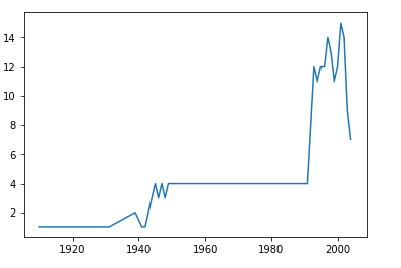﻿ Pandas Datetime: Create a plot to present the number of unidentified flying object (UFO) reports per year - w3resource# Pandas Datetime: Create a plot to present the number of unidentified flying object (UFO) reports per year

## Pandas Datetime: Exercise-7 with Solution

Write a Pandas program to create a plot to present the number of unidentified flying object (UFO) reports per year.

Sample Solution :

Python Code :

``````import pandas as pd
df['Date_time'] = df['Date_time'].astype('datetime64[ns]')
print("Original Dataframe:")
print("\nPlot to present the number unidentified flying objects (ufo) found year wise:")
df["Year"] = df.Date_time.dt.year
df.Year.value_counts().sort_index().plot(x="Year")
``````

Sample Output:

```Original Dataframe:
Date_time                  city     ...       latitude   longitude
0 1910-06-01 15:00:00           wills point     ...      32.709167  -96.008056
1 1920-06-11 21:00:00                cicero     ...      40.123889  -86.013333
2 1929-07-05 14:00:00  buchanan  (or burns)     ...      43.642500 -118.627500
3 1931-06-01 13:00:00               abilene     ...      38.917222  -97.213611
4 1939-06-01 20:00:00              waterloo     ...      34.918056  -88.064167

[5 rows x 11 columns]

Plot to present the number unidentified flying objects (ufo) found year wise:```

Python Code Editor:

Have another way to solve this solution? Contribute your code (and comments) through Disqus.

What is the difficulty level of this exercise?

﻿

## Python: Tips of the Day

Python: Use Enumerate() In for Loops

```>>> students = ('John', 'Mary',  'Mike')
>>> for i, student in enumerate(students):
...     print(f'Iteration:  {i}, Student: {student}')
...
Iteration: 0, Student: John
Iteration: 1, Student: Mary
Iteration: 2, Student: Mike
>>> for i, student in enumerate(students,  35001):
...      print(f'Student Name: {student}, Student ID #: {i}')
...
Student Name: John, Student ID #: 35001
Student Name: Mary, Student ID #: 35002
Student Name: Mike, Student ID #: 35003
```